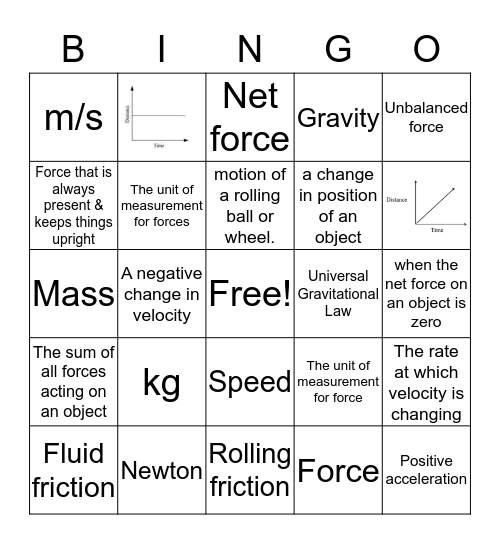# Motion & Forces BINGOThis bingo card has 3 images, a free space and 42 words: Acceleration, Positive acceleration, Negative acceleration, Fluid friction, Sliding Friction, Force, Net force, Balanced Force, Unbalanced force, Newton, Gravity, Universal Gravitational Law, Speed, kg, Friction, Rolling friction, Static friction, Mass, Distance, Velocity, m/s, A push or a pull, when the net force on an object is zero, the property of matter that measures its resistance to acceleration, velocity at a specific time 't', The unit of measurement for force, The sum of all forces acting on an object, An objects resistance to change its motion, The rate at which velocity is changing, Magnitude of distance traveled divided by time taken, speed + direction, The quantity of matter in an object, A negative change in velocity, An objects resistance to change in its motion, A force that counteracts motion, A positive change in velocity, motion of a rolling ball or wheel., Force that is always present & keeps things upright, a change in position of an object, the resistance force that slows down the, The unit of measurement for forces and the frictional force that prevents two surfaces from sliding past each other.

## Play Online